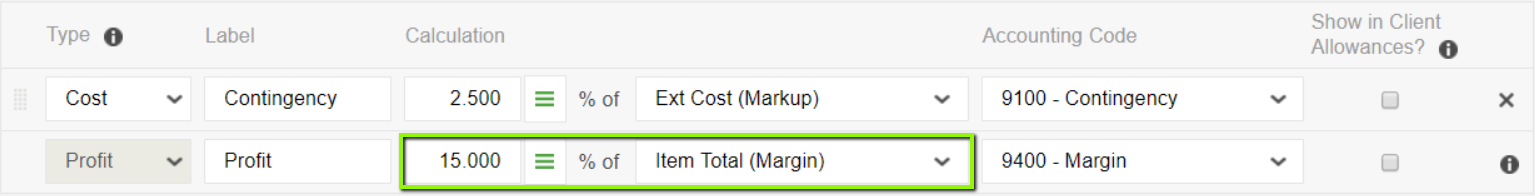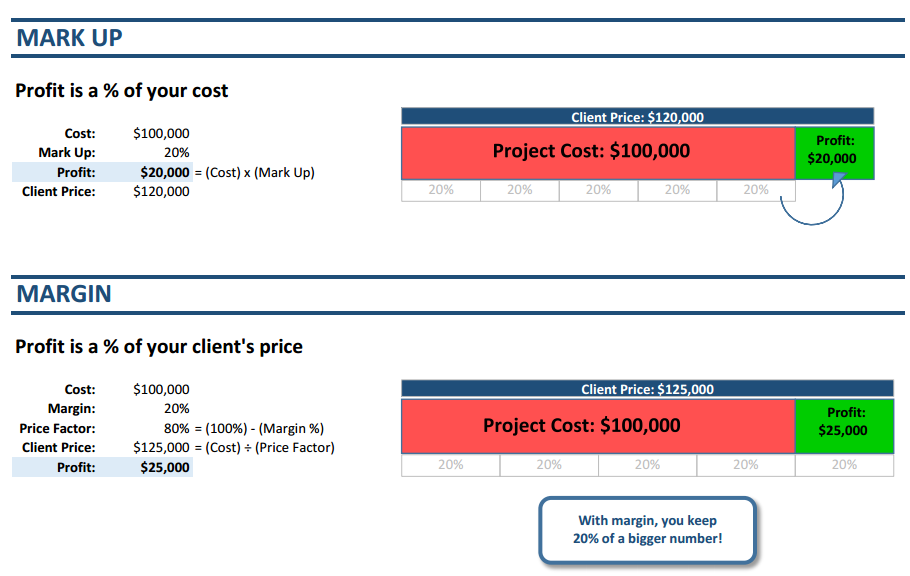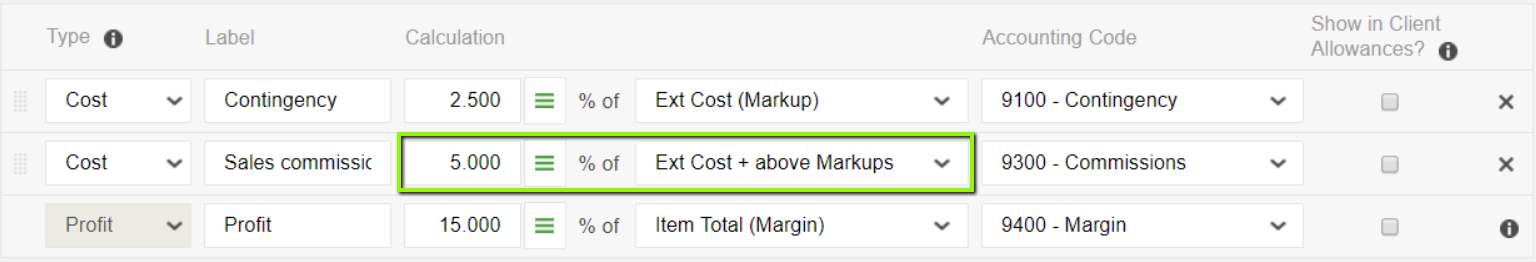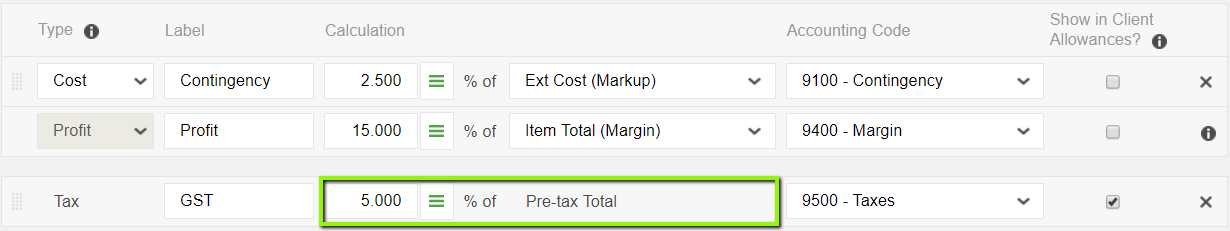Follow

# How are markup, margin, and tax calculated?

11

In CoConstruct, not only can you add markup throughout your estimates, but you have the flexibility to specify your default markup amounts in a few unique ways.

% of Ext Cost (Markup)

Your markup will be calculated as a percentage of the extended cost for each line. For projects where markup is configured globally, in rows at the bottom of the estimate, this percentage will be applied to the subtotal for the project.

Example CalculationIn the screenshot above, a 2.500% contingency markup is applied to the base costs of each estimate line.

If the extended cost (quantity multiplied by unit cost) is \$100, then the contingency markup would be calculated in the following way:

• 2.500% x \$100 = \$2.50

% of Item Total (Margin)

This will base your markup on the total, or the final price after markup for the line item.

Example CalculationIn the example above, Profit is calculated to be 15.000% of the total price.

The math gets increasingly complicated with the more markups you have at once, but as a basic example, here's how the margin calculation would look for a \$100 extended cost:

• Total = Ext Cost + Contingency + Profit
• Total = (\$100.00 + \$2.50 + (15.000% x Total))
• Total = (\$100.00 + \$2.50) / (1 - 15.000%) = \$120.59
• In the example above, a \$100 cost would result in a \$120.59 total for the line item.
• That total will leave 15.000% x \$120.59 = \$18.09 allocated to Profit.

Note that if we instead calculated profit as 15% of the "Ext Cost", then that profit would be closer to 12-13% of the overall marked up total.

By building your profit as a percent of the total (margin) rather than a percent of the cost (markup), you'll always know what percentage of the final price will go to you.

Markup vs. Margin: Understanding the difference% of Ext Cost + above Markups

If you have more than markup calculations set up, you will see an additional option, for "% of Ext Cost + above Markups."

You may think of this as a calculation on a running total, where markup is calculated based on the sum of the cost and any previous markup amounts on the line.

In your Markup/Margin page, this will calculate the percentage on top of all markups that are higher in the list, but will ignore markups that are further down in the list.

Example CalculationIn the screenshot above, a sales commission markup is applied as 5.000% on the extended cost, plus all of the above markups, which includes the calculated contingency amount. Profit is not marked up by the 5.000% commission markup, because it is listed below the tax markup.

If the extended cost is \$100, then the markup calculation for this sales commission would be:

• 5.000% x (Extended Cost + Contingency)
• 5.000% x (\$100 + \$2.50) = \$5.13

Fixed amount

If you're handling your markup as rows rather than columns, you also have the ability to set a fixed markup amount that will mark up the subtotal of all of your estimate lines.

This option is not available when your markup is configured as columns, since the markups apply to each and every line on your estimate, rather than just to the subtotal.

% of Pre-Tax Total (Tax calculations only)

If you add a tax calculation on the Markup, Margin, and Tax page, your tax will be calculated on top of the pre-tax project total (after any prior markup/margin calculations are applied).

Example CalculationIn the screenshot above, a 5% Goods and Services Tax (GST) is applied to the cost after a 2.5% contingency and a 15% margin are accounted for.

From the above Margin example calculation, the pre-tax total for a \$100 base cost would be \$120.59.

GST would be calculated as:

• 5.000% x (Pre-tax Total)
• 5.000% x \$120.59 = \$6.03

So for a base cost of \$100, the price for the client after factoring in a 2.5% contingency (\$2.50), a 15% margin (\$18.09), and a 5% sales tax (\$6.03) would be \$126.62.